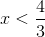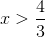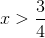Example Questions

Example Question #5 : How To Find A Rational Number From An Exponent

Simplify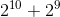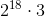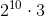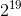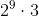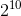Explanation:

Whenever you see lots of multiplication (e.g. exponents, which are notation for repetitive multiplication) separated by addition or subtraction, a common way to transform the expression is to factor out common terms on either side of the + or - sign. That allows you to create more multiplication, which is helpful in reducing fractions or in reducing the addition/subtraction to numbers you can quickly calculate by hand as you'll see here.

So let's factor a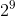.

We have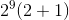.

And you'll see that the addition inside parentheses becomes quite manageable, leading to the final answer ofExample Question #1 : How To Find Out When An Equation Has No Solution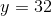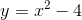Quantity A: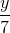Quantity B:Quantity B is greater.

The two quantities are equal.

Quantity A is greater.

The relationship cannot be determined from the information given.

The relationship cannot be determined from the information given.

Explanation:

We are given that y = 32.  Plug this value of y into the second equation.

32 = x2 – 4

36 = x2

x = +/– 6.

Next find a value for Quantity A:

y/7 = 32/7

This number is less than +6, but more than –6. Thus, the relationship cannot be determined from the information given.

Example Question #41 : Gre Quantitative Reasoning

Column A: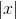Column B: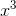The values are equal.

The relationship cannot be determined.

Column B is greater.

Column A is greater.

The relationship cannot be determined.

Explanation:

Column B is greater for positive numbers.

The columns are equal for 0.

Column A is greater for negative numbers.

Because our answer changes depending on the value inserted, we cannot determine the relationship.

Example Question #1 : Linear / Rational / Variable Equations

Find the solution to the following equation if x = 3:

y = (4x2 - 2)/(9 - x2)

6

0

no possible solution

3

no possible solution

Explanation:

Substituting 3 in for x, you will get 0 in the denominator of the fraction. It is not possible to have 0 be the denominator for a fraction so there is no possible solution to this equation.

Example Question #1 : How To Find Out When An Equation Has No Solution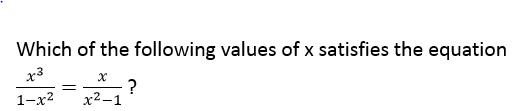I.  x = 0

II. x = –1

III. x = 1

I only

III only

I, II, and III

II only

II and III only

I only

Explanation: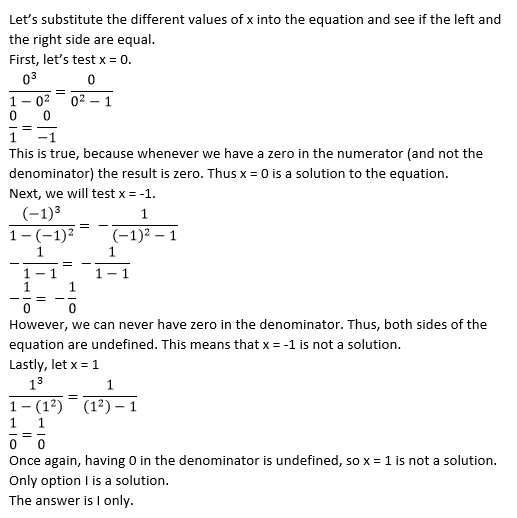Example Question #1 : How To Find Out When An Equation Has No Solution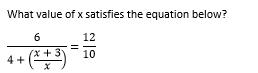1

–1/2

–3

3

There is no solution

There is no solution

Explanation: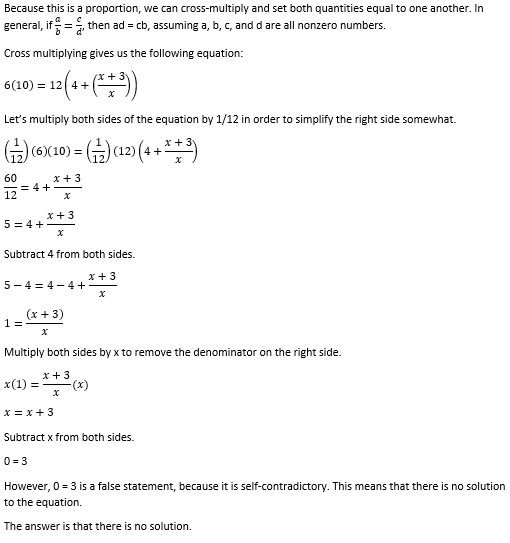Example Question #1 : Equations / Inequalities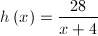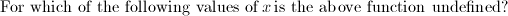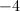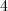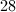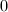Explanation:

A fraction is considered undefined when the denominator equals 0. Set the denominator equal to zero and solve for the variable.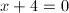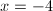Example Question #10 : How To Find Out When An Equation Has No Solution

Solve: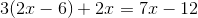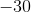Explanation:

First, distribute, making sure to watch for negatives.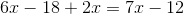Combine like terms.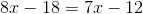Subtract 7x from both sides.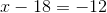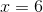Example Question #3 : How To Find Out When An Equation Has No Solution

Solve: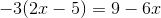Infinitely Many Solutions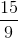No Solution

No Solution

Explanation:

First, distribute theto the terms inside the parentheses.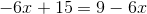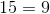This is false for any value of. Thus, there is no solution.

Example Question #6 : Linear / Rational / Variable Equations

Solve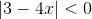.

No solutions